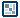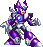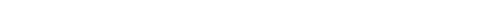## Question of the WeekBinary-Decimal Conversion
by Zelwyn : 22 December 2004

In this tutorial, I'm going to introduce you to the binary number system, if you don't already know it, and teach you to convert a decimal number into binary and vice versa using a little bit of code in Flash. First of all, I assume all of you know what the decimal number system is. It's the one we use everyday! It's base number is ten, meaning (basically) that when you get past nine in one place, you increase the next place value by one, and also that each place value is equal to the one before it multiplied by ten. Now, binary is a little different.

When we talk about binary, we are talking about the "1's and 0's" language you think about if you're a big computer geek (no offense). Your computer is made up of a whole lot of switches, each triggering and action in your computer when flipped. A 1 in binary symbolizes an "on" in the computer, whereas a 0 symbolizes an "off". The binary number system has a base number of two, meaning that each place is equal to the one before it times two. So that being said, if the first digit (the far right) is 1, then they go in this order from right to left: 1, 2, 4, 8, 16, 32, 64, 128, 256, and so on.

 256, 128, 64, 32, 16, 8, 4, 2, 1

[ an example of the order of places ]

Here is How you convert normally
Here I'll teach you how to look at any binary number and convert it into decimal.

010011110

This number is equal to 158. How did I get that? Well, if you follow the table that I showed you in the beginning, the first digit is 1, followed by 2, and so on. Each place where there is a one, you add the number in that place to your total. So, since there's a 0 in the "1's" place, we don't add 1, but since there's a 1 in the "2's" place, we will add 2. There's a 1 in each of these places: 2, 4, 8, 16, and 128, so, 2+4+8+16+128=158. That's so easy isn't it? Here, try this one: 101010101. By the way, when doing this while at the computer, the scientific mode of Windows Calculator has a feature to convert between hexadecimal, decimal, octal, and binary. You should have gotten 341 if you did it right. That was easy wasn't it? To convert from decimal is much harder. You take the next lowest place value from your number (unless your number is the same as a place value, such as 32), and you divide your number by that place, take the remainder, rinse and repeat until you get to 1. So, if our number was 100, we would divide it by 64, and get 36. We would then divide that by 32, and get 4. We divide 4 by 4, and get 1. Then we make our number and put a 1 in every place that we divided by. So, 100 in binary is 1100100.

Here is How you convert in Flash
Here I'll teach you how to convert any binary number to decimal and vice versa, in Flash.

To convert from binary to decimal, just like in real life, is much easier to do in Flash than the other way around. Add this code into the first frame of your movie:

What this does is it makes two arrays that correspond the binary number to the place values that it goes with. It then multiplies each value by the value in the binary array, and adds them together. You could make a text field display them. Now add this code for the conversion the other way around:

// convert decimal number to binary string
function dec2bin(iNumber) {
var bin = "";
var oNumber = iNumber;
//this while method constructs a string from the number you enter as iNumber when you call the function
while (iNumber>0) {
if (iNumber%2) {
bin = "1"+bin;
} else {
bin = "0"+bin;
}
iNumber = Math.floor(iNumber/2);
}
/*while (bin.length<15) {
bin = "0"+bin;
}*/
return bin;
}

What this does is adds a 1 and 0 for each place where they're needed. If you uncomment the while method in there, it'll add zeroes until it's a certain length, determined by the "15" in that while statement. That should be good for conversion of numbers. I might make one on octal or hexadecimal eventually.

For any of you that want to learn about other number systems I would suggest a book called How to count like a martian by Glory St. John, and Cool Math by Christy Maganzini and Ruta Daugavietis. They helped me a lot. Anyone interested in binary might also want to check out my Binary Clock. If you can't read it go to this one: Binary Clock. You can probably expect me to make tutorials on other systems sooner or later. Cya!

Peace be with you...Zelwyn